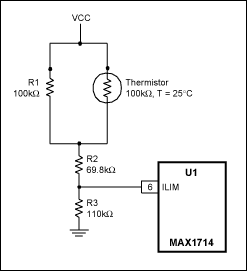# Thermistor Linearizes Current Limit

### Abstract

A resistive network that includes a thermistor is used to temperature-compensate the current-limit input (ILIM) of a DC-DC converter.

Recent advances in the design of step-down DC-DC converters have eliminated the current-sense resistor by substituting the drop across the low-side MOSFET (synchronous rectifier) instead. This topology saves the cost and space of a sense resistor, and also provides a modest boost in efficiency. One compromise imposed by the new approach, however, is a current-limit value dominated by the MOSFET's on-resistance, which is highly temperature dependent.

Fortunately, the new DC-DC converters provide a pin that allows adjustment of the current-limit threshold. By changing this threshold according to temperature, you can temperature-compensate the circuit's output-current limit. That task is readily accomplished with a thermistor as shown in Figure 1.The linear input range for the ILIM input of U1 is 0.5V to 2.0V, which corresponds to current-limit thresholds of 50mV to 200mV, respectively. For the default current-limit setting (100mV), the circuit has a 7.5A current limit at +25°C. As shown in Figure 2, however, the limit ranges from 9A at -40°C to 6A at +85°C.The intent of this design is to cancel U1's temperature variation using a thermistor-based compensation circuit. Figure 1 depicts one of several possible resistor/thermistor topologies. First, choose and characterize the thermistor. R1 serves to linearize the thermistor, while R2 and R3 are chosen so that the slope and intercept of VILIM versus temperature directly compensate for temperature variations in the current limit.

The curves shown in the corrected output characteristic (Figure 2) are intrinsic to the thermistor. Though not perfectly flat, the corrected slope is a great improvement over the original and sufficient for our purpose. (You can achieve a more precise compensation using a different thermistor or multiple thermistors.) This circuit provides higher current limits at room temperature or colder, while meeting its specification at higher temperatures.

This article appeared as a Design Idea in the October 13, 2005 edition of EDN magazine.